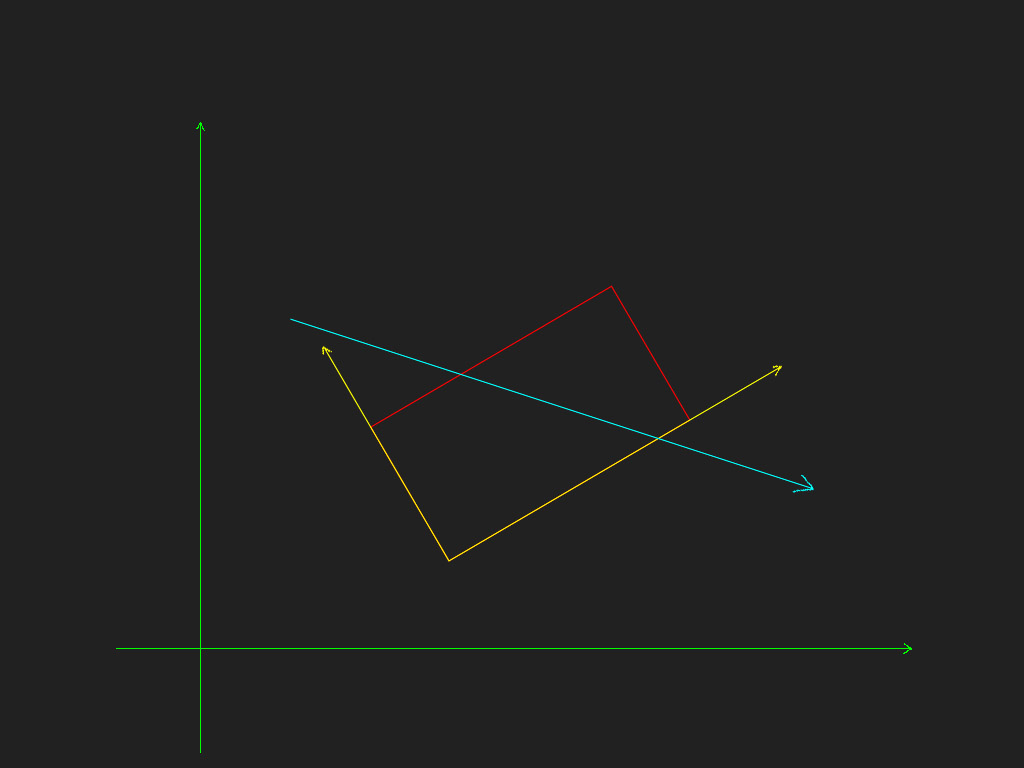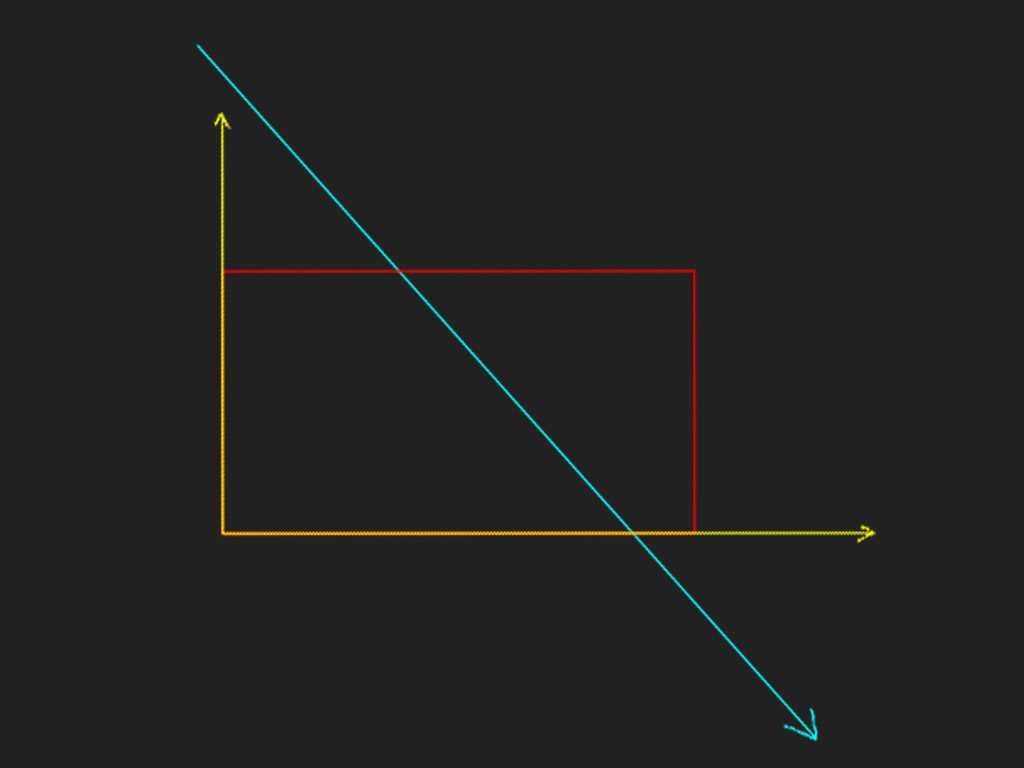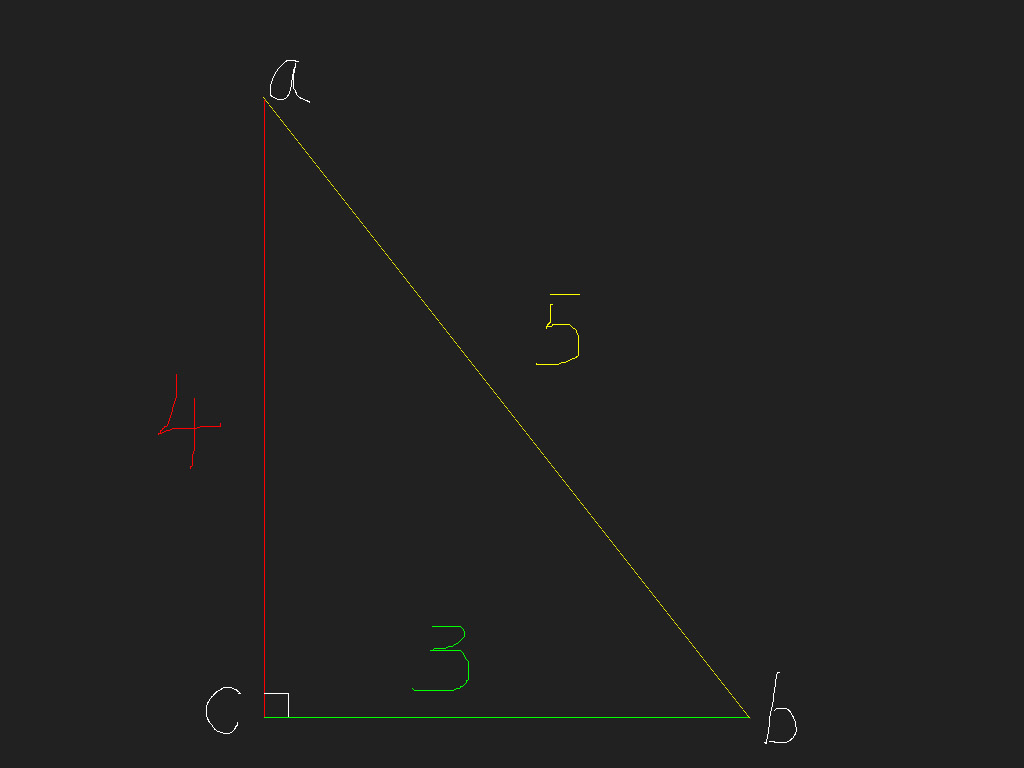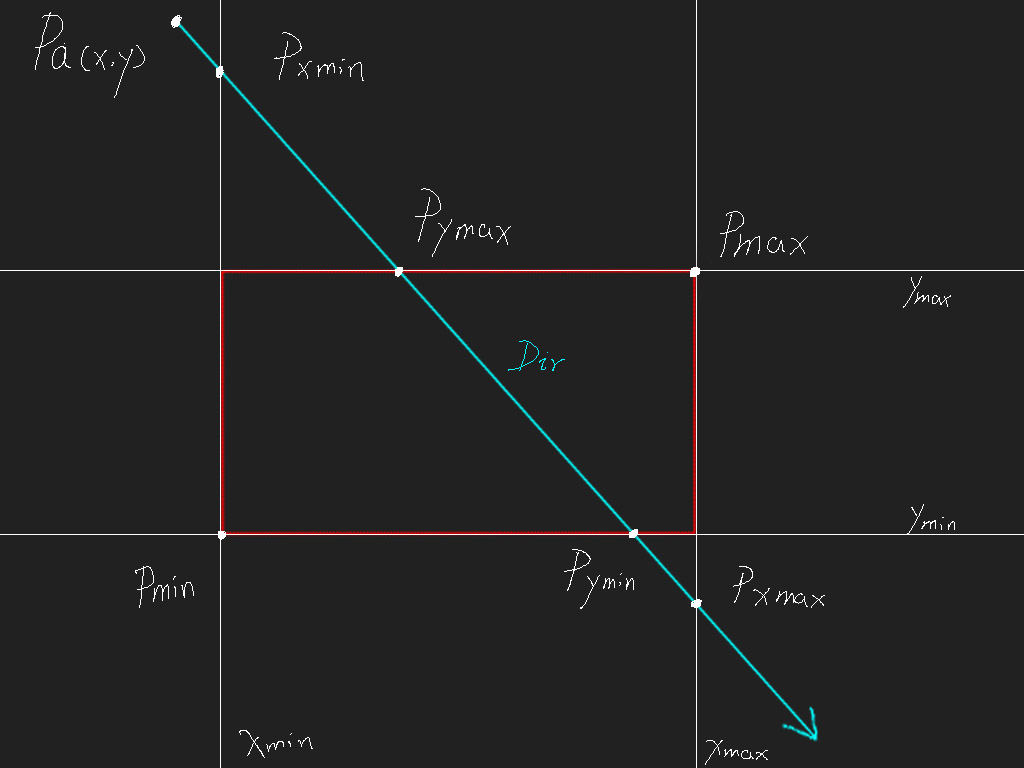# Jim's GameDev Blog# 矩形射线相交检测

2016-3-8``````黄色边长 = 绿色边长 / normalize(向量ab).x

````````````txmin = (Xmin - Pa.x) / Dir.x
``````

``````tymax = (Ymax - Pa.y) / Dir.y
``````

``````tymin = (Ymin - Pa.y) / Dir.y
``````

``````txmax = (Xmax - Pa.x) / Dir.x
``````

``````Pxmin = Pa + Dir * txmin
``````